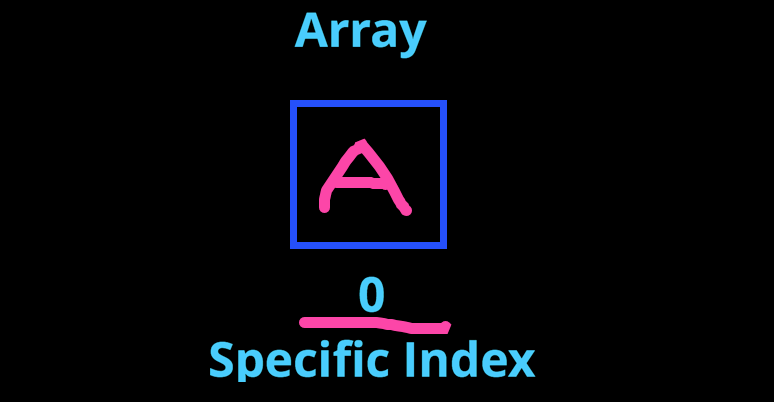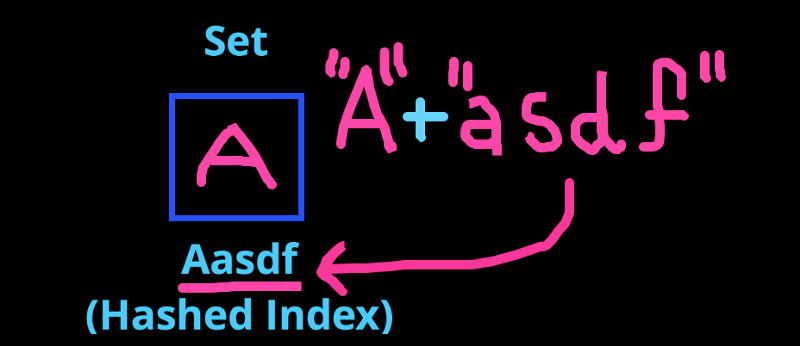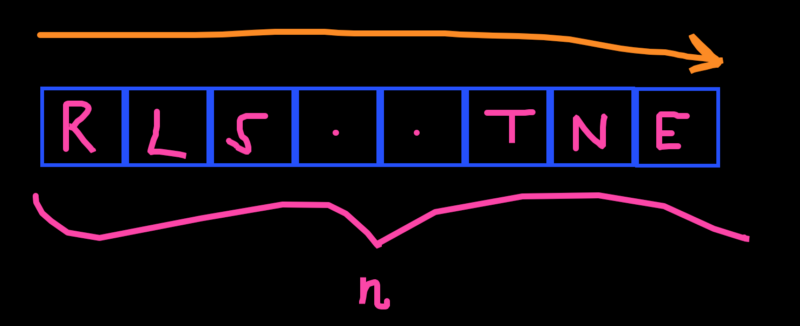by Anthony Sistilli

# Learn your coding fundamentals: the main differences between sets and arrays

A question I get a lot from my CS students at The Forge is why I often use sets instead of plain old arrays in interview problems.

To answer that question, we have to understand the fundamental differences between a set and an array.

If you are a visual learner and prefer a video explanation, here’s a 3 minute video that explains the answer (albeit in less depth).

Arrays were one of the first data structures I learned how to use.

Not only are they a fundamental data structure used in almost every coding application, but they’re fairly easy to understand as well.

It wasn’t until way later in my software career that I got introduced to the array’s strange, but magical, cousin:

The Set.

Sets are like arrays… except they’re not.

#### Let’s quickly remind ourselves about how an array works

Arrays:

• Are ordered
• Have indices starting at 0
• Can contain duplicate elements
• Have a O(n) lookup time when you search for an element

#### However, sets behave a bit differently

Sets:

• Are unordered (in almost all languages)
• Have hashed indices
• Can NOT contain duplicate elements
• Have a O(1) lookup time when searching for an element

Let’s take a more in-depth look.

### 1. Sets Insert By Hashing

The elements in a set are stored quite differently than that of an array.

The way a set stores its elements is by Hashing.

#### Let’s say you want to store the character “A” in a set and an array.

The array would simply find the next available index, unless otherwise specified, and place the element in that index.Our “A” gets an index of 0 since it’s the first element.

With hashing, however, things look a bit different.

#### How Hashing Works

Hashing is the act of taking in input (x), distorting it with a specific hash function (h), and getting a final output (y).

Basically h(x) = (y)

Looks a bit confusing right?

Don’t worry! This should clear things up.

An easy example of a hashing function (h) could be appending “asdf” to the end of your input (x).

If (x) is “A” and appending “asdf” is (h), the output (y) would simply be as follows:

“A” + “asdf” →“Aasdf”

So “Aasdf” would be our (y).

#### So, how does a set use Hashing?

A set uses hashing to decide where to store your input (x).

In a nutshell, a set takes your input, hashes it, and stores it at the index that matches the hashed input, AKA the output (y).Aasdf is the index of our element “A”.

This is the reason why sets are unordered in most languages.

Array indexing is easy, 0 to n, so you can easily remember what comes next.

But with the complex hashing functions most compilers use, the order that the elements were inserted in can’t be found unless you keep a secondary indexing mechanism.

### 2. Sets Can Not Contain Duplicates

That’s right!

A set can only contain unique elements.

Contrary to how it sounds, this can actually be extremely helpful in a lot of situations, including Google Interview Questions.

Why does it do that, you ask?

Well, because of hashing!

Since my hashing function (h) will remain consistent as my program runs, inputting the same (x) will always give you the same (y).

That means if I tried to insert a second “A”, my hashing function would output the same address as the first “A”, and it would simply overwrite it!

With an array, it would simply append the second “A” to the next available index.

### 3. Sets Have An O(1) Lookup Time

Let’s say you have an array of n elements, where n is a big number, and you wanted to see if “A” existed in that array.

Well, worst case scenario, “A” doesn’t exist.

And to find that out, you would have to iterate through all n of those elements!ARRAY. OF. FORTUNE!

That gives an Array a time complexity of O(n) when it comes to looking up an element.

#### We can save a lot of time with a Set

If we wanted to find whether or not an element exists in our set, all we have to do is hash that element and check the index!

Remember: The index an element is stored in is connected to the element itself.

Therefore, if we wanted to see whether or not “A” existed in our set, we would just have to hash it (+ “asdf”) and check that index!

Since this process will always take a constant amount of operations, no matter how big the set is, it has a constant time complexity.

That means a set has a time complexity of O(1) when it comes to looking up an element… Which is a huge improvement!

### Can you think of any situations where this is useful?

If you can’t, check out this Google Interview Question where a set makes all the difference!

Thanks for reading!

.a

P.S — For more data structures & algorithms tutorials, and interview prep, check out www.TheForge.ca!

We help students and new grads land their dream software job!